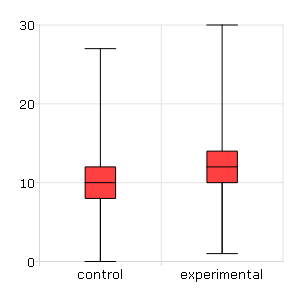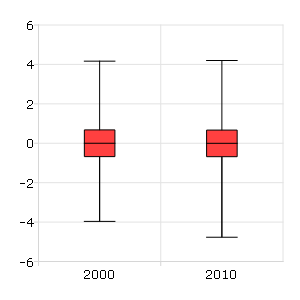# plotBox¶

## Purpose¶

Graphs data using the box graph percentile method.

## Format¶

plotBox([myPlot, ]group_id, y)
plotBox([myPlot, ]df_groups, y)
plotBox([myPlot, ]df, formula)
Parameters
• myPlot (struct) – Optional argument, a plotControl structure

• group_id (Mx1 vector or Mx1 string array) – This contains the group numbers or string labels corresponding to each column of y data. If scalar 0, a sequence from 1 to cols(y) will be generated automatically for the x-axis.

• y (NxM matrix) – Each column represents the set of y values for an individual percentiles box symbol.

• df_groups (Nx1 categorical dataframe variable) – A categorical dataframe vector. The y variable will be split by the categories in df_groups to create the boxes to graph.

• df (dataframe) – name of the dataframe in memory.

• formula (string) –

formula string of the model to be plotted. E.g "y ~ X1", y is the name of dependent variable to be plotted on the y-axis X1 is the names of the variable to be plotted on the x-axis;

E.g "y ~ X1 + by(X2)", by(X2) specifies that the data should be separated into different lines based on the groups defined by X2.

## Examples¶

### Dataframe with formula string¶

// Import data
fname = getGAUSSHome() $+ "examples/auto2.dta"; auto2 = loadd(fname); // Draw a box with 'mpg' data for each of // the two categories in 'foreign' plotBox(auto2, "mpg ~ foreign");  ### Dataframe with formula string¶ // Import data fname = getGAUSSHome()$+ "examples/tips2.dta";

// Draw a box with 'tip' data for each day,
// split by whether 'smoker' equals yes or no.
plotBox(tips, "tip ~ day + by(smoker)");


### Matrix data with string labels¶

// Create two columns of random data
nobs = 1e5;
y_1 = rndPoisson(nobs, 1, 10);
y_2 = rndPoisson(nobs, 1, 12);

// Create a 2x1 string array, using the
// horizontal string concatenation operator
labels = "control" \$| "experimental";

// Draw the two boxes
plotBox(labels, y_1~y_2);### Matrix data with numeric labels¶

// Create two columns of random data
nobs = 1e5;
y_1 = rndn(nobs, 1);
y_2 = rndn(nobs, 1);

// Create a 2x1 vector of numeric labels
labels = { 2000, 2010 };

// Draw the two boxes
plotBox(labels, y_1~y_2);## Remarks¶

Note that numeric labels added to the x-axis are only labels for the corresponding boxes. They do not imply any order or denote a particular x-axis location.

If missing values are encountered in the y data, they will be ignored during calculations and will not be plotted.